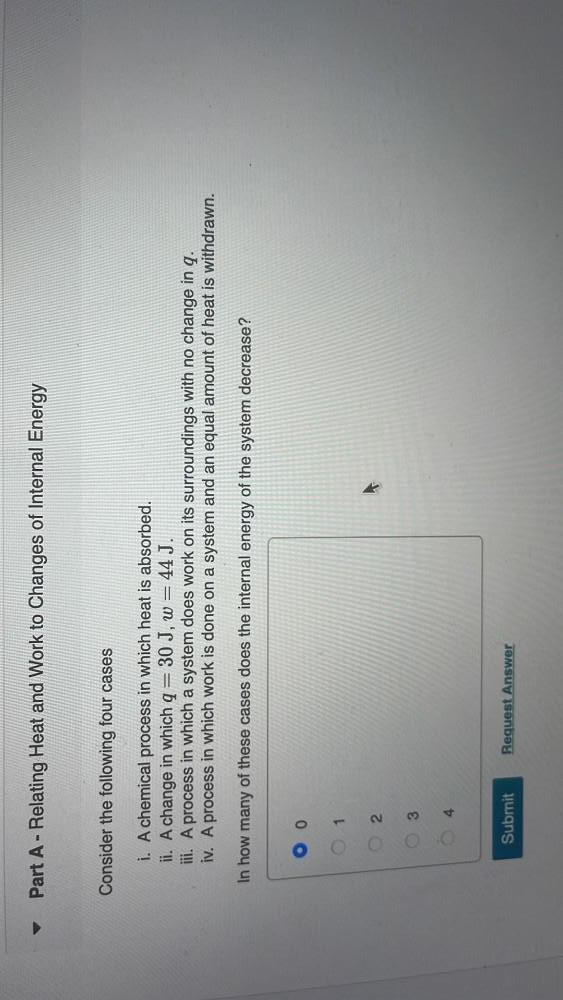Question:

# Consider the following four cases i. A chemical process in which heat is absorbed. ii. A change in which q = 30 J, w = 44 J. iii A process in which a system does work on its surroundings with no changConsider the following four cases i. A chemical process in which heat is absorbed. ii. A change in which q = 30 J, w = 44 J. iii A process in which a system does work on its surroundings with no change in q. iv. A process in which work is done on a system and an equal amount of heat is withdrawn. In how many of these cases does the internal energy of the system decrease? O 0 O 1 O 2 O 3 O 4